Home > CC3 > Chapter 9 > Lesson 9.1.4 > Problem9-48

9-48.
1.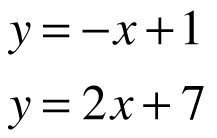Solve the system of equations at right by each of the ways described in parts (a) and (b) below. Then compare your answers in part (c). Homework Help ✎

1. Graph the system on graph paper. Then write its solution (the point of intersection) in (x, y) form.

2. Now solve the system using the Equal Values Method.

3. Did your solution in part (b) match your result from part (a)? If not, check your work carefully and look for any mistakes in your algebraic process or on your graph.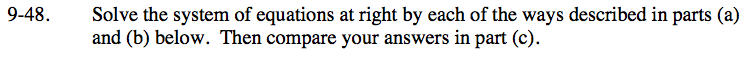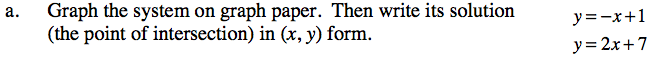When the equation of a line is written in y = mx + b form, the coefficient of the x(m) represents the slope, while the other number (b) represents the y-intercept.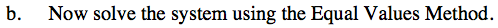The first step in the Equal Values method is to set the equations equal to each other.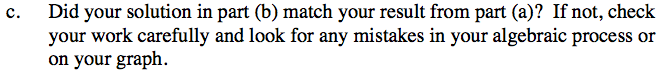(−2, 3) Your answers should be the same for parts (a) and (b).